output.to from Sideway

Sound in Enclosure 3D

Draft for Information Only

# Content

``` Wave Reflection inside Enclosures, 3D   Number of Modes ```

## Wave Reflection inside Enclosures, 3D

The 3D wave equation is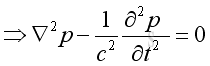Assume harmonic sound wave imply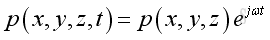Take the time differential operation, imply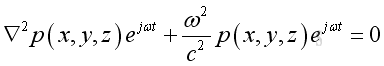And get the Helmoltz equation,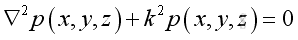Assume the pressure is of format: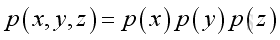substitute the pressure function into the helmoltz equation, and take the Laplacian differential operation: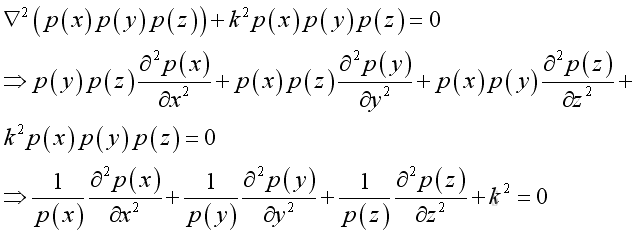let the dispersion equation :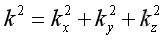substitute into the Helmoltz equation: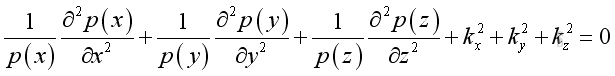By separating the variable, imply: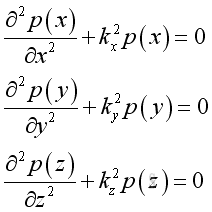Assume the corresponding solution of the equation of the form,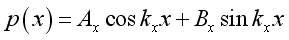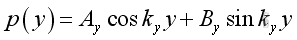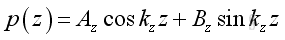Assume the rectangular enclosure are with rigid walls, the boundary conditions at 6 walls are with particle velocity equals to zero, imply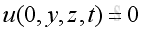,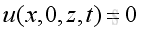,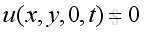and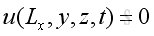,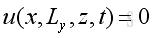,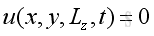From the equation of momentum conservation in x direction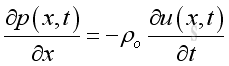At x = 0 or x=Lx, u=0 at all time, therefore the time derivative of u is zero also, imply: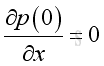and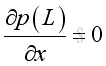Therefore at x=0 and x=Lx, imply :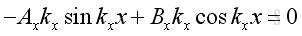From boundary condition at x=0, imply: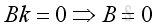From boundary condition at x=Lx, imply:Substitute kx, A and B into the pressure function, imply:and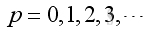Similarly ky, kz,are: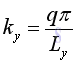and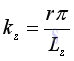And the corresponding pressure function in y and z direction are: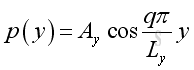and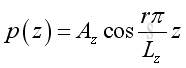, where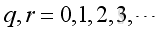Substitute all individual pressure functions into the total pressure function is: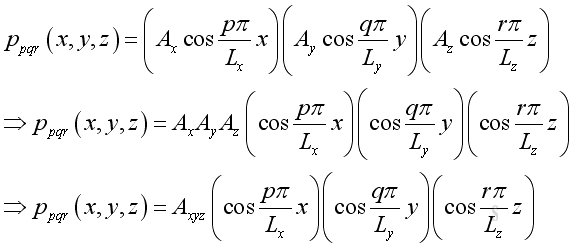where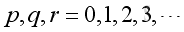Substitute all individual wave number into the combined wave number is: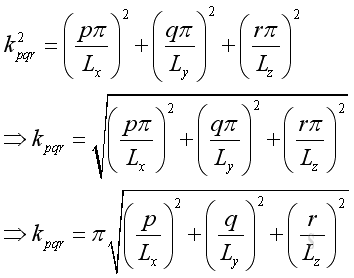whereTherefore the frequency is :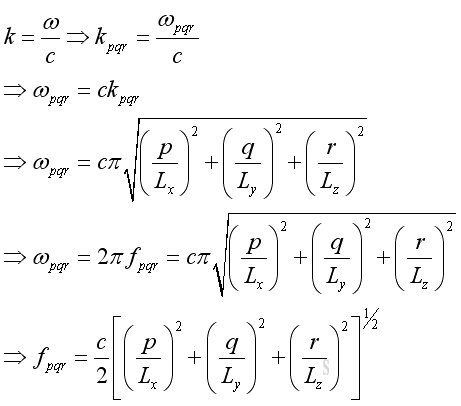where### Number of Modes

The number of modes increases dramatically with the increase of frequency and with the volume of the cavity and can be estimated by.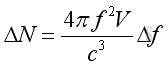References

1. Michael P. Norton; Denis G. Karczub,, 2003, Fundamentals of Noise and Vibration Analysis for Engieer, Cambridge, United Kingdom
2. G. Porges, 1977, Applied Acoustics, Edward Arnold Limited, Britain
3. Douglas D. Reynolds, 1981, Engineering Principles of Acoustics:; Noise and Vibration Control, Allyn and Bacon, USA
4. Conrad J. Hemond, 1983, Engineering Acoustics & Noise Control, Prentice-Hall, USA
5. F. Fahy, 2001, Foundations of Engineering Acoustics, Academic Press, UK
6. D.A. Biew; C.H. Hansen, 1996, Engineering Noise Control: Theory and Practice, E & FN Spon, New YorkID: 101000022 Last Updated: 10/20/2010 Revision: 0 Ref:Home (1)

Management

HBR (3)

Information

Recreation

Hobbies (7)

Culture

Chinese (1097)

English (334)

Reference (65)Computer

Hardware (148)

Software

Application (187)

Digitization (24)

Numeric (19)

Programming

Web (540)CSS (SC)

HTML

Knowledge Base

Common Color (SC)

Html 401 Special (SC)

OS (367)

MS Windows

Windows10 (SC)

DeskTop (5)

Knowledge

Mathematics

Formulas (8)

Number Theory (206)Algebra (17)

Trigonometry (18)

Geometry (18)

Calculus (67)

Complex Analysis (5)Engineering

Tables (8)

Mechanical

Mechanics (1)

Rigid Bodies

Statics & Dynamics (129)

Fluid (5)

Control

Acoustics (19)

Biology (1)

Geography (1)